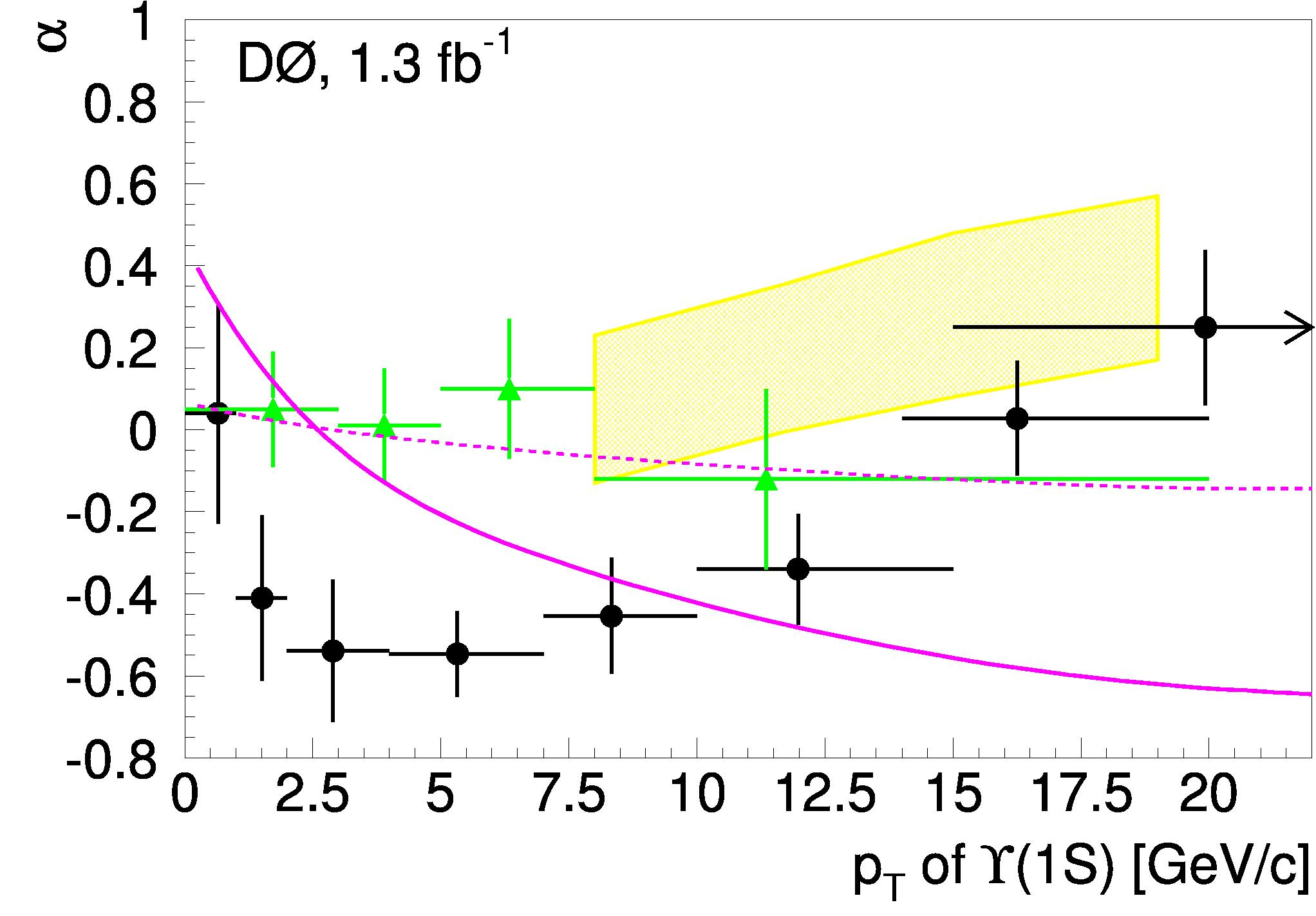## Upsilon polarization: a surprise from D0August 27, 2008

Posted by dorigo in news, physics, science.
Tags: , , , , , ,

I was surprised by the recent measurement by the D0 collaboration of the Upsilon polarization, which finds a sizable effect which really disagrees with the CDF result, obtained six years ago and based on a 12 times smaller dataset from Run I.

D0 has a large acceptance to muons, and so can detect with good efficiency the$\Upsilon(1S) \to \mu^+ \mu^-$ decays. CDF has a slightly worse acceptance, but its momentum resolution is of a totally different class. Compare the mass distribution of Upsilon mesons published by D0 in their polarization paper, shown below (they refer to different bins of transverse momentum, left to right, and to different fit parametrizations, top to bottom; black points are the data, the red curve is the fit, and the black gaussians are the three Upsilon signals returned by the fit),…with the Run I distribution by CDF on which their old result is based, in the plot below (where the data is the black histogram, and the curve is the fit):Any questions ? Of course, the three Upsilon(1S), (2S), (3S) states merge together in a broad bump in the D0 signal plot, while they stand each on their own in the CDF plot. That’s resolution, baby. Muon momentum resolution is a thing on which Nobel prizes are done and undone.

Despite the lower resolution, D0 can statistically separate the three populations, and measure the Upsilon (1S) and (2S) polarization as a function of the meson transverse momentum. The polarization is defined by the number alpha:$\large \alpha = \frac {\sigma_T-2 \sigma_L} {\sigma_T+2 \sigma_L}$,

where$\sigma_T$ and$\sigma_L$ are the cross-sections for producing a transversely and longitudinally-polarized meson, respectively. The polarization can be measured from the decay angle of the positively-charged muon in the Upsilon rest frame.

D0 had a total of 260,000 Upsilon decays to play with, and they produced a very detailed measurement of the behavior of$\Upsilon(1S)$ and$\Upsilon(2S)$ polarization as a function of the meson$P_T$. This allows a comparison with NRQCD, a factorization approach to the calculation of quarkonium production processes which enshrines in universal non-perturbative color-octet matrix elements the non-computable part, and uses experimental data to fix them.

Confused? Don’t be. Let’s just say that NRQCD is a successful approach at determining several characteristics of charmonium production, and a test of its prediction of the dominance of$\sigma_T$ at high transverse momentum -where gluon fragmentation is the main process for the production of quarkonium in the model- is quite useful.

Another thing to note is that understanding Upsilon production -particularly in the forward region- may be very important for the determination of parton-distribution functions of the proton at very small values of Bjorken x -the fraction of proton momentum carried by a parton. These measurements are very important for the LHC, where interesting physics phenomena will be dominated by very low x collisions.

So let me just jump to the results of the D0 analysis. The polarization plot for the 1S state is shown below. Black points are the D0 measurement, while the green ones show the old CDF result (by the way, it is a shame that CDF does not have a Run II measurement of the Upsilon polarization yet, and you can well say it is partly my fault, since a few years ago I wanted to do this measurement and then had to abandon it…).As you can see, the D0 result shows a Pt dependence of polarization which is not well matched by the NRQCD predictions (the yellow band, which is only available above 8 GeV of Pt), nor by the two limiting cases of a kt-factorization model. What is worse, however, is that the result comes seriously at odds with the old CDF data points. Who is right and who is wrong ? Or are the two sets of points compatible ?

Well, this is one of those instances when counting standard deviations does not work. The two results have sizable correlated systematic uncertainties among the data points, so moving eight data points up by one sigma collectively may cost much less than$\sqrt{8}$ standard deviations: in the limit that the systematics dominate and they are 100% correlated, moving all points up by$1 \sigma$ just costs one standard deviation… In the case of the D0 points, however, one does not have this information from the paper. One learns that the signal modeling systematics amount to anywhere between 1 and 15%, with the bin with maximum uncertainty being the second one from left; and that background modeling systematics range from 4 and 21%, with the first bin being the worst one. As for the old CDF result, I could not find a detailed description of systematics either, but in that case the precision of the measurement is driven by statistics.

In any case, congratulations to D0 for producing this important new measurement. And I now hope CDF will follow suit with their large dataset of Upsilons too!